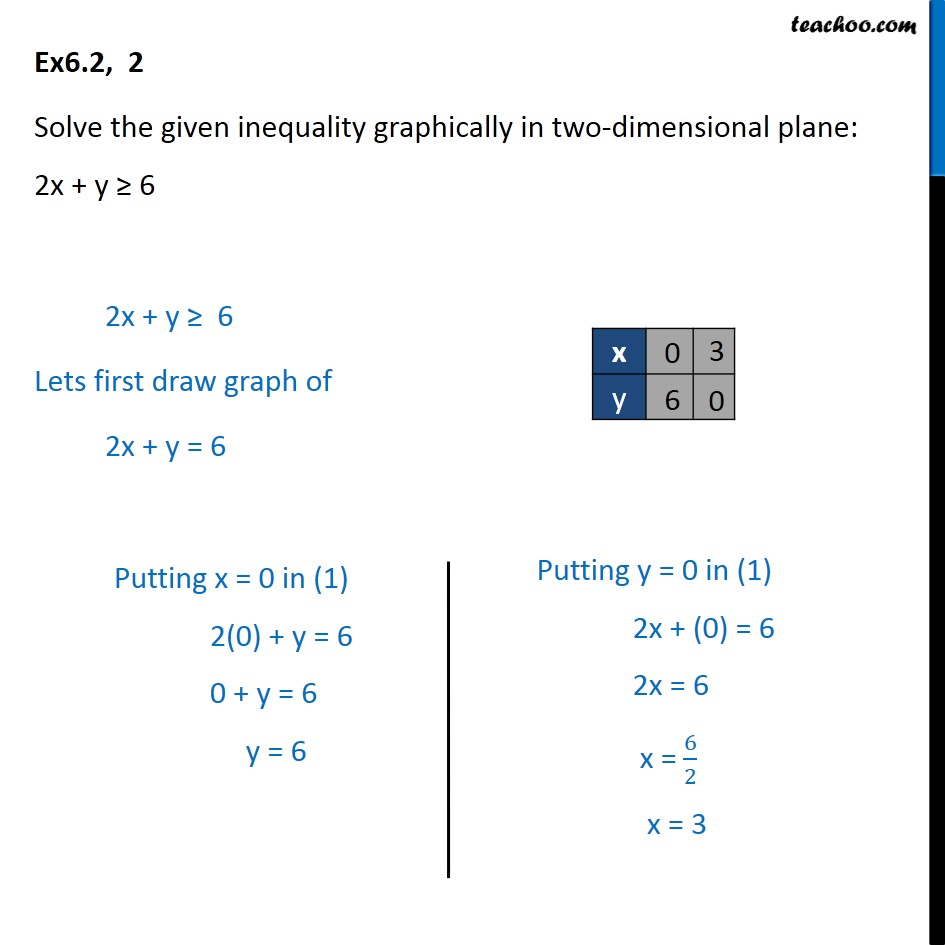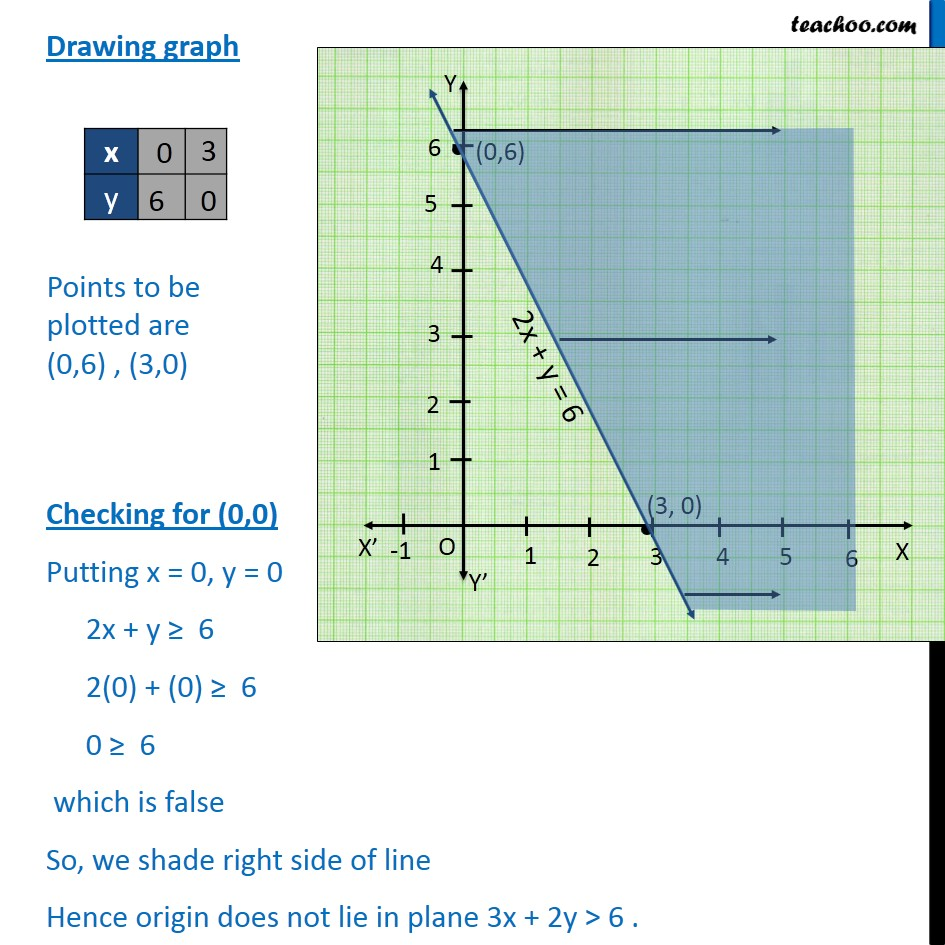1. Chapter 6 Class 11 Linear Inequalities (Term 2)
2. Serial order wise
3. Ex 6.2

Transcript

Ex6.2, 2 Solve the given inequality graphically in two-dimensional plane: 2x + y 6 2x + y 6 Lets first draw graph of 2x + y = 6 Drawing graph Checking for (0,0) Putting x = 0, y = 0 2x + y 6 2(0) + (0) 6 0 6 which is false So, we shade right side of line Hence origin does not lie in plane 3x + 2y > 6 .